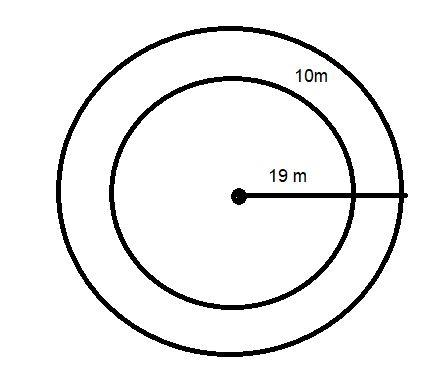Courses
Courses for Kids
Free study material
Free LIVE classes
More# Find the circumference of the inner and the outer circles, shown in the adjoining figure. (Take π=3.14)Verified
326.4k+ views
Hint: Here we go through by applying the formula of circumference of a circle i.e. $2\pi r$ because the radius is given in the question’s figure. For inner circumference use inner radius and for outer circumference use outer radius.

$\therefore$ Circumference of outer circle $= 2\pi R = 2 \times 3.14 \times 19 = 119.32m$
$\therefore$Circumference of inner circle$= 2\pi r = 2 \times 3.14 \times 9 = 56.52m$